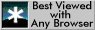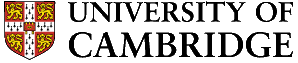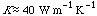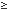# AP4, Examples Class

Start with Qu.1 or Qu.2, as directed. The class takes up the first 40 minutes of the practical period.

1. A zinc die casting is to be produced with a maximum section thickness of 8 mm. The casting must be ejected from the die 4 seconds after the liquid charge has been injected. The die remains at 100°C throughout and the liquid is injected without superheat. Estimate the minimum heat transfer coefficient required at the die/casting interface if the casting is to be fully solid when ejected. Comment on the practical feasibility of achieving the required value.

[For Zn, the fusion temperature is 420°C, the latent heat of fusion is 500,000,000 J m-3, thermal conductivity,]

2. The carburization of steels is an important example of a surface-hardening process. Since the surface concentration of carbon is held constant as the carbon diffuses into the steel, the error function solution to the diffusion equation is applicable. In this question we solve this equation using the MATTER software. Open the MATTER module on Atomic Diffusion in metals and Alloys - Interstitial Diffusion, and turn to p.14. Assume that the initial concentration of carbon in the steel is 0.2 wt.%, and that the surface concentration is 0.8 wt.%. Set these values (under ‘Options’), and a temperature of close to 1100°C. Select the plotting interval ‘small (smoother)’. Plot.

(a) What is the diffusion coefficient of carbon?

(b) After 1000 s of carburization what depth of material has a carbon content0.6 wt.%?

(c) After 1000 s of carburization what depth of material has a carbon content0.4 wt.%?

(d) A sample given this carburizing treatment is air-cooled to room temperature. Indicate, using sketches, how the microstructures near the surface of the sample and in the bulk would differ.

[This examples class is followed, within the normal practical period, by an assessed practical lasting one hour. The assessed practical is based on AP1, AP2, AP3, and this examples class; it includes a small amount of practical work and some usage of the MATTER software.